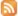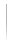know more about 2020 nike and adidas soccer cleats news,check shopcleat and wpsoccer.

know more about 2020 nike and adidas soccer cleats news,check shopcleat and wpsoccer.

# Sizing Guidelines for MatrixAir™ Building-Integrated Solar Air Heating Systems

##### Calculating the AREA of collector surface needed according to total CFM required for façade mounted systems :
###### Transpired solar air heating collector MatrixAir™(TR)
• Total CFM required divided by 3 = Maximum total ft2 of collector required.
• Total CFM required divided by 6 = Average total ft2 of collector required.
• Total CFM required divided by 9 = Minimum total ft2 of collector required.
###### Backpass solar air collector * MatrixAir™(BP)
• Total CFM required divided by 35 = Maximum number of 12 inch wide modules
• Total CFM required divided by 60 = Average number of 12 inch wide modules
• Total CFM required divided by 90 = Minimum number of 12 inch wide modules
• * MatrixAir™ BP collector should be a minimum of 10 ft and no more than 34 ft high.
##### Calculating the AREA of collector surface needed according to total CFM required for roof mounted systems :
###### Delta Roof top MatrixAir™ (DT)
• Total CFM required divided by 250 = Number of modules required.
##### Calculating the CFM that may be produced according to available Wall area for façade mounted systems
###### Transpired solar air heating collector MatrixAir™ (TR)
• Total wall area (ft2) multiplied by 9 = Maximum recommended CFM delivered
• Total wall area (ft2) multiplied by 6 = Average recommended CFM delivered
• Total wall area (ft2) multiplied by 3 = Minimum recommended CFM delivered
###### Backpass solar air collector MatrixAir™ (BP)
• One half of the overall wall width (in feet) multiplied by 180 = Maximum recommended CFM delivered
• One half of the overall wall width (in feet) multiplied by 150 = Average recommended CFM delivered
• One half of the overall wall width (in feet) multiplied by 100 = Minimum recommended CFM delivered
##### Calculating the CFM that may be produced according to available Roof area for roof mounted systems
###### Delta roof mounted collector * MatrixAir™ (DT)
• Total roof area (ft2) multiplied by 4.4 = Maximum CFM delivered (for most North American locations).
• * Based on multiple row configurations. Use a factor of 6.6 for single row installations.Tweet
About UsSite MapPrivacy PolicyContact UsMatrix Energy